## HTML5创意画板的设计教程

1 评论 1.5万 浏览 2 收藏 20 分钟```　　document.addEventListener('mousemove', mouseMove, false);

`　　setInterval(loop, 1000 / 60);`
```function loop() {
\$pos_display.innerHTML='你当前鼠标的位置为('+pos.x+','+pos.y+')';
if (isMouseDown) draw(context);}```

loop为循环执行的函数。

```function mouseDown(e) {
isMouseDown = true;
}```

```function loop() {
if (isMouseDown) draw(context);//绘制鼠标点击位置
}```
```function mouseMove(e) {
pos.x=e.clientX;//设置x坐标
pos.y=e.clientY;//设置y坐标
\$pos_display.innerHTML='你当前点击鼠标的位置为('+pos.x+','+pos.y+')';//更新当前鼠标点击的位置
}```

```function draw(ctx) {

ctx.save();//保存当前绘图状态
ctx.fillStyle = DEFAULT_BRUSH_COLOR;//设置填充的背景颜色
ctx.lineWidth =DEFAULT_BRUSH_SIZE;  //设置画笔的大小
ctx.lineCap = "round"; //设置线条，让线条边缘更圆滑
ctx.beginPath();
ctx.arc(pos.x,pos.y,DEFAULT_BRUSH_SIZE,0,Math.PI * 2,true);
/****
*context.arc(x, y, radius, startAngle, endAngle, anticlockwise)
*参数 x,y表示圆心
*startAngle起始弧度
*endAngle终止弧度
*anticlockwise是否为逆时针方向
***/
ctx.fill();//填充绘画路径
ctx.restore();//恢复绘画状态

}```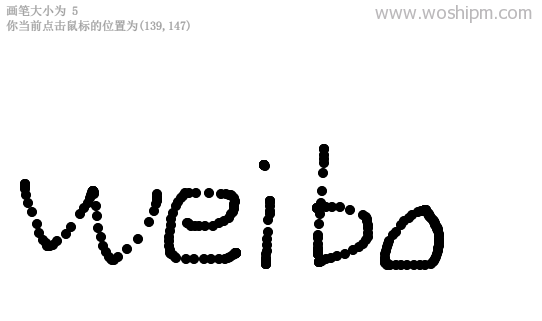`　　setInterval(loop, 1000 / 60);`

`　　setInterval(loop, 1000 / 1000);`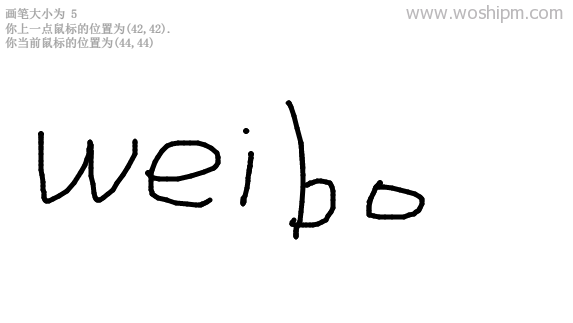```if(pos.x==next_pos.x&&pos.y==next_pos.y){
ctx.arc(pos.x,pos.y,DEFAULT_BRUSH_SIZE/1.7,0,Math.PI * 2,true);
ctx.fill();//填充绘画路径}```
```else{
ctx.moveTo(pos.x,pos.y);
ctx.lineTo(next_pos.x,next_pos.y);
ctx.stroke();
}```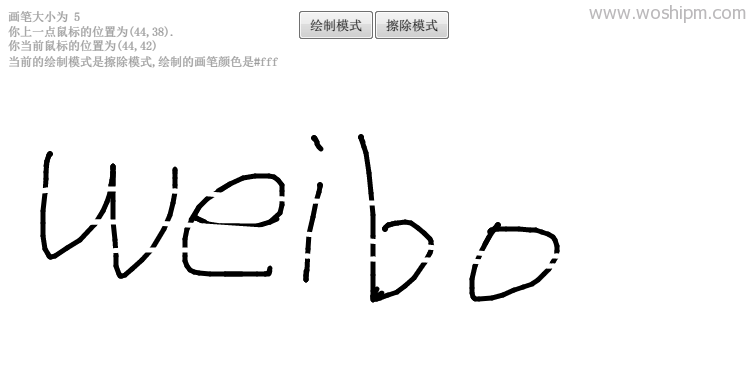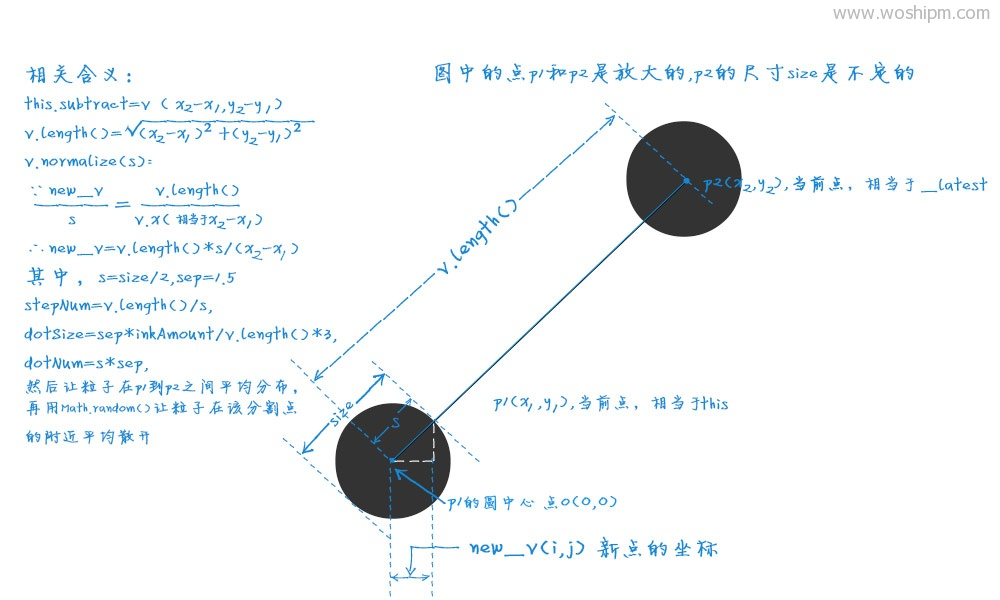```　　draw: function(ctx) {
var v = this.subtract(this._latest);//当前点与下一个点的距离的横纵坐标
var s = Math.ceil(this.size / 2);		//算出粒子的单位长度
var stepNum = Math.floor(v.length() / s) + 1;	//算出步长  v.length()为斜线长度
v.normalize(s);//当前点与下一个点的

var sep = 1.5; // 分割数  控制画笔的浓密程度  关键所在
//粒子的大小 根据画笔描绘的速度（画笔的停留时间）进行调整
var dotSize = sep * Math.min(this.inkAmount / this._latestStrokeLength * 3, 1);
var dotNum = Math.ceil(this.size * sep);
var range = this.size / 2;
var i, j, p, r, c, x, y;
\$whitemode_display.innerHTML="绘制的画笔颜色是"+brush_color;
ctx.save();
ctx.fillStyle = currentColor;
\$pos_display.innerHTML='你上一点鼠标的位置为('+this.x+','+this.y+').

ctx.beginPath();
if(wmode=="擦除模式"){
ctx.strokeStyle=brush_color;
ctx.lineWidth =DEFAULT_BRUSH_SIZE;
ctx.lineCap = "round";
ctx.beginPath();
p = this._latest;//获取下一个点位置
ctx.moveTo(this.x,this.y);
ctx.lineTo(p.x,p.y);
ctx.stroke();
}
else{
for (i = 0; i < dotNum; i++) {
for (j = 0; j < stepNum; j++) {
r = random(range);
c = random(Math.PI * 2);
w = random(dotSize, dotSize / 2);
h = random(dotSize, dotSize / 2);
x = p.x + r * Math.sin(c) - w / 2;
y = p.y + r * Math.cos(c) - h / 2;
ctx.rect(x, y, w, h);//边缘不要太平滑，不要使用arc
}
}
}
ctx.fill();
ctx.restore();
}
});```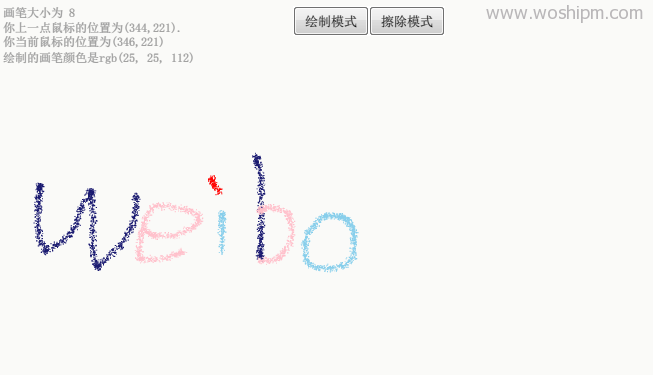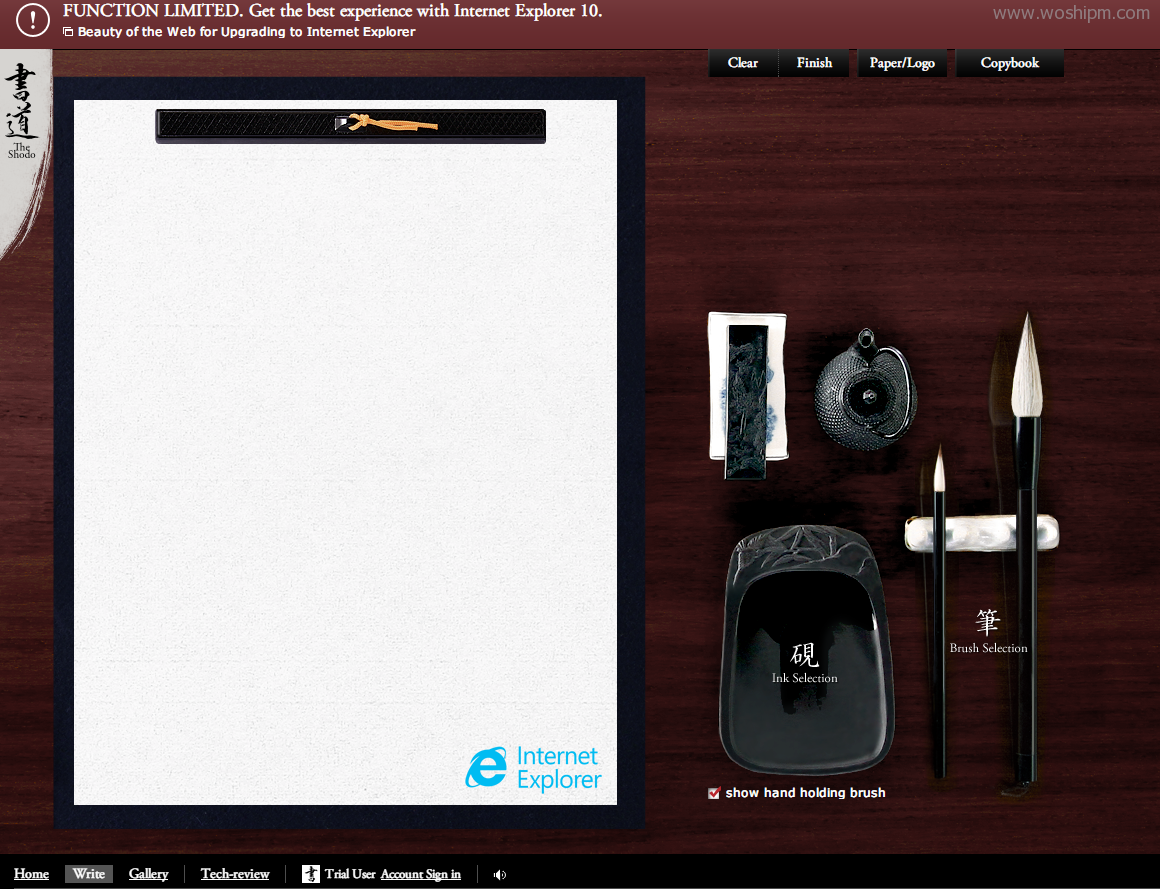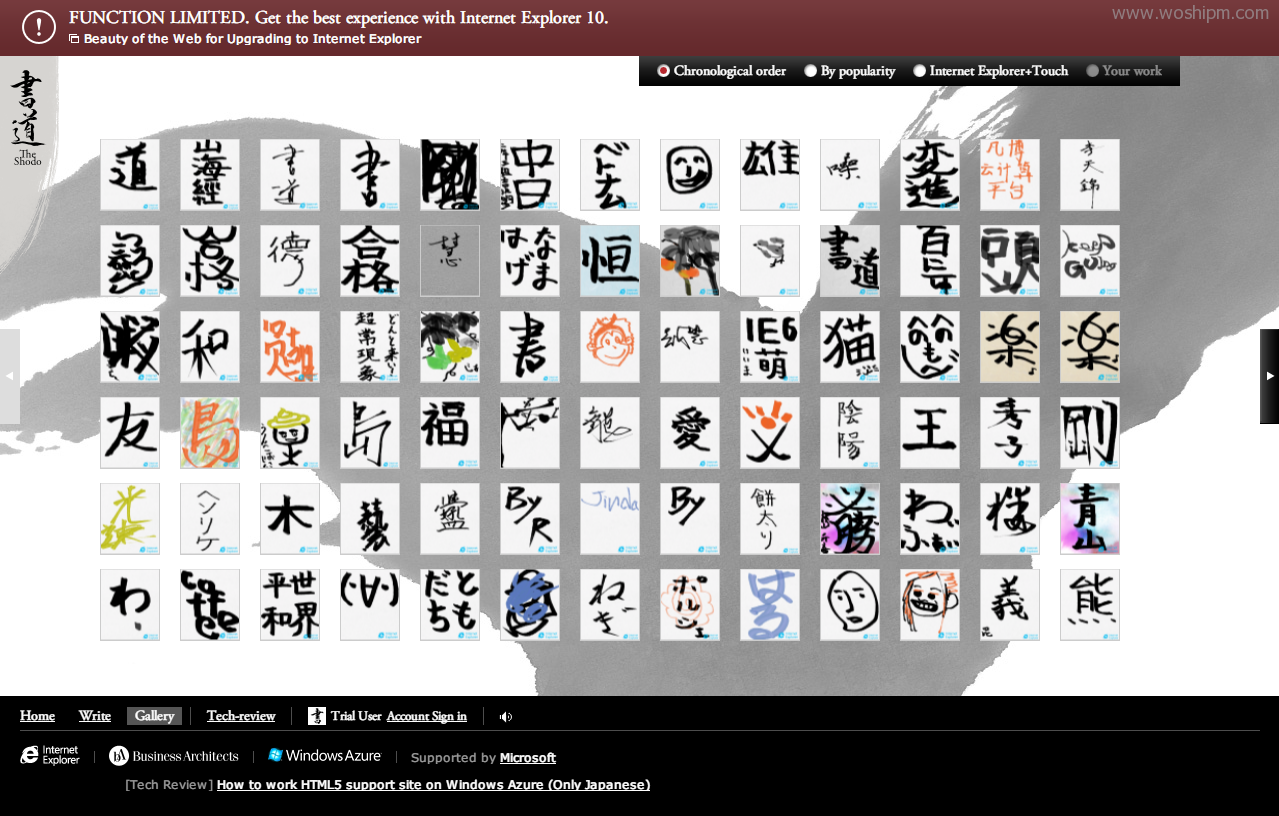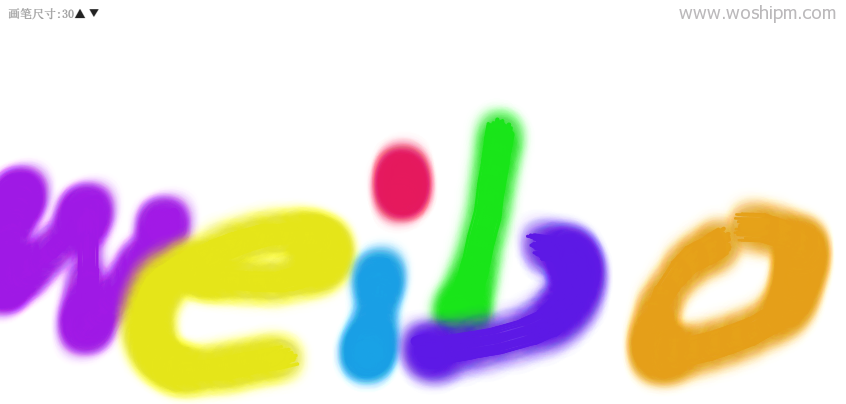runJS: http://runjs.cn/detail/spxs2kxq

1. 请问DEMO6如何改成可以在移动端上玩，我试过把mousedown,mouseup,mousemove,改成touchstart,touchmove,touchend也不行 🙁

回复